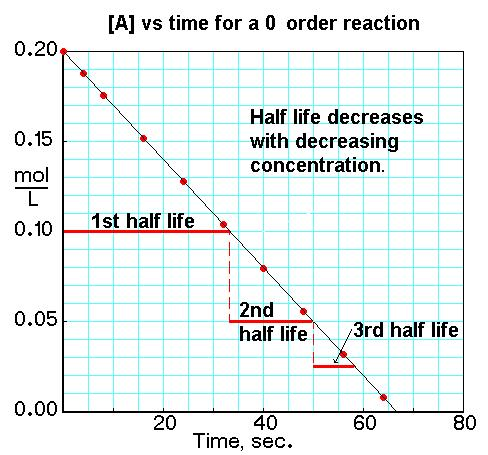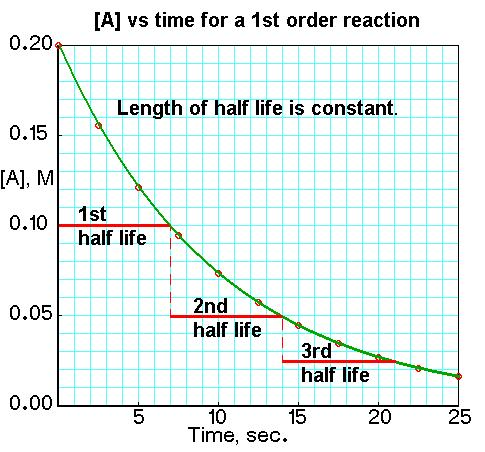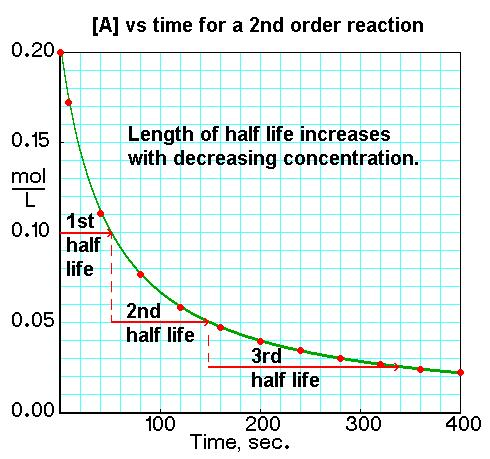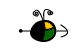Half Lives

We use integrated rate laws, and rate constants to relate concentrations and time. The rate law to use depends on the overall order of the reaction.

Equations for Half Lives

For a zero order reaction  Aproducts   ,    rate =  k:

t½ =  [Ao] / 2k
For a first order reaction      Aproducts  ,   rate =  k[A]:
t½ = 0.693 / k
For a second order reaction   2Aproducts   or   A + Bproducts   (when [A] = [B]),      rate = k[A]2:
t½ = 1 / k [Ao]

Top

Determining a Half Life

To determine a half life, t½, the time required for the initial concentration of a reactant to be reduced to one-half its initial value, we need to know:

• The order of the reaction or enough information to determine it.
• The rate constant, k, for the reaction or enough information to determine it.
• In some cases, we need to know the initial concentration, [Ao]

Substitute this information into the equation for the half life of a reaction with this order and solve for t½. The equations are given above.

Top

Converting a Half Life to a Rate Constant

To convert a half life to a rate constant we need to know:

• The half life of the reaction, t½.
• The order of the reaction or enough information to determine it.
• In some cases, we need to know the initial concentration, [Ao]

Substitute this information into the equation for the half life of a reaction with this order and solve for k. The equations are given above.

Top

Graphical Relations and Half Lives

If we plot the concentration of a reactant versus time, we can see the differences in half lives for reactions of different orders in the graphs. We can identify a 0, 1st, or 2nd order reaction from a plot of [A] versus t by the variation in the time it takes the concentration of a reactant to change by half.

For a zero order reaction      Aproducts   ,    rate =  k:For a first order reaction      Aproducts  ,   rate =  k[A]:For a second order reaction   2Aproducts   or   A + Bproducts   (when [A] = [B]),        rate = k[A]2: